Hello, Guest!
 Instructional Focus DocumentAlgebra II
 TITLE : Unit 13: Exploring a Business Venture SUGGESTED DURATION : 10 days

Unit Overview

Introduction
This is a project-based unit where students apply bundled student expectations that address collecting, representing, and analyzing data to develop a platform for a business venture in order to make predictions and defend decisions in terms of the business situation. According to the Texas Education Agency, mathematical process standards including application, tools and techniques, communication, representations, relationships, and justifications should be integrated (when applicable) with content knowledge and skills so that students are prepared to use mathematics in everyday life, society, and the workplace.

Prior to this Unit
In Algebra I, students investigated linear functions, equations, and inequalities and systems of two linear equations in two variables. Students also investigated quadratic functions and equations and exponential functions. In Algebra II, students investigated quadratic, rational, and exponential functions and equations. Students solved systems of three linear equations in three variables, systems of at least two linear inequalities in two variables, and a system involving a linear equation and quadratic equation.

During this Unit
Students apply prior knowledge to investigate and plan business ventures. Student groups explore possible scenarios for business ventures and each group selects a business venture to develop. Students represent at least two different data sets involved in the business venture that can be modeled by linear, quadratic, exponential, inverse variation, or other appropriate functions. Students identify characteristics of the functions, and analyze various aspects involving their business venture in terms of the representative functions, equations, inequalities, and systems of equations and inequalities. Students create a final written summary of their business venture, including appropriate diagrams, displays, algebraic generalizations, and calculations, justifying all solutions and conclusions in terms of their business venture. Students then analyze and critique other business venture reports.

After this Unit
In subsequent courses in mathematics and later careers, these concepts will continue to be applied to problem situations involving functions, equations, inequalities, and systems of equations and inequalities.

In Algebra II, applying functions, equations, inequalities, and systems of equations and inequalities to model real-world situations are identified in STAAR Readiness Standards 2A.3A, 2A.3B, 2A.4F, 2A.5D, 2A.6L, and 2A.8C and STAAR Supporting Standards 2A.3C, 2A.3E, 2A.3G, 2A.4E, and 2A.5B. These standards are subsumed under STAAR Reporting Category 2: Describing and Graphing Functions and Their Inverses, STAAR Reporting Category 3: Writing and Solving Systems of Equations and Inequalities, STAAR Reporting Category 4: Quadratic and Square Root Functions, Equations, and Inequalities, STAAR Reporting Category 5: Exponential and Logarithmic Functions and Equations, and STAAR Reporting Category 6: Other Functions, Equations, and Inequalities. This unit is supporting the development of Texas College and Career Readiness Standards (TxCCRS): I. Numeric Reasoning A2, B1; II. Algebraic Reasoning A1, B1, C1, D1, D2; III. Geometric Reasoning C1; VII. Functions A2, B1, B2, C1, C2; VIII. Problem Solving and Reasoning; IX. Communication and Representation; X. Connections.

Research
According to the National Council of Teachers of Mathematics (2000), Principles and Standards for School Mathematics, students should develop an understanding of the algebraic properties that govern manipulation of symbols in expressions, equations, and inequalities. According to Navigating through Algebra in Grades 9 – 12, “High school students continue to develop fluency with mathematical symbols and become proficient in operating on algebraic expressions in solving problems. Their facility with representation expands to include equations, inequalities, systems of equations, graphs, matrices, and functions, and they recognize and describe the advantages and disadvantages of various representations for a particular situation. Such facility with symbols and alternative representations enables them to analyze a mathematical situation, choose an appropriate model, select an appropriate solution method, and evaluate the plausibility of their solutions,” (NCTM, 2002, p. 3). Research found in National Council of Teachers of Mathematics also states, “Using a variety of representations can help make functions more understandable to a wider range of students than can be accomplished by working with symbolic representations alone,” (NCTM, 2009, p. 41). This unit places particular emphasis on multiple representations. State and national mathematics standards support such an approach. The Texas Essential Knowledge and Skills repeatedly require students to relate representations of functions, such as algebraic, tabular, graphical, and verbal descriptions. This skill is mirrored in the Principles and Standards for School Mathematics (NCTM, 1989). Specifically, this work calls for instructional programs that enable all students to understand relations and functions and select, convert flexibly among, and use various representations for them. More recently, the importance of multiple representations has been highlighted in Curriculum Focal Points for Prekindergarten through Grade 8 Mathematics (NCTM, 2007). According to this resource, students should be able to translate among verbal, tabular, graphical, and algebraic representations of functions and describe how aspects of a function appear in different representations as early as Grade 8. Also, in research summaries such as Classroom Instruction That Works: Research-Based Strategies for Increasing Student Achievement, concept development is even cited among strategies that increase student achievement. Specifically, classroom use of multiple representations, referred to as nonlinguistic representations, and identifying similarities and differences has been statistically shown to improve student performance on standardized measures of progress (Marzano, Pickering & Pollock, 2001).

Marzano, R. J., Pickering, D. J., & Pollock, J. E. (2001). Classroom instruction that works: Research-based strategies for increasing student achievement. Alexandria, VA: Association for Supervision and Curriculum Development.
National Council of Teachers of Mathematics. (2000). Principles and standards for school mathematics. Reston, VA: National Council of Teachers of Mathematics, Inc.
National Council of Teachers of Mathematics. (2002). Navigating through algebra in grades 9 – 12. Reston, VA: National Council of Teachers of Mathematics, Inc.
National Council of Teachers of Mathematics. (2007). Curriculum focal points for prekindergarten through grade 8 mathematics. Reston, VA: National Council of Teachers of Mathematics, Inc.
National Council of Teachers of Mathematics. (2009). Focus in high school mathematics: Reasoning and sense making. Reston, VA: National Council of Teachers of Mathematics, Inc.
Texas Education Agency & Texas Higher Education Coordinating Board. (2009). Texas college and career readiness standards. Retrieved from http://www.thecb.state.tx.us/index.cfm?objectid=E21AB9B0-2633-11E8-BC500050560100A9

 Quantitative relationships model problem situations efficiently and can be used to make generalizations, predictions, and critical judgements in everyday life. What patterns exist within different types of quantitative relationships and where are they found in everyday life? Why is the ability to model quantitative relationships in a variety of ways essential to solving problems in everyday life? Statistical displays often reveal patterns within data that can be analyzed to interpret information, inform understanding, make predictions, influence decisions, and solve problems in everyday life with degrees of confidence. How does society use or make sense of the enormous amount of data in our world available at our fingertips? How can data and data displays be purposeful and powerful? Why is it important to be aware of factors that may influence conclusions, predictions, and/or decisions derived from data?
Unit Understandings
and Questions
Overarching Concepts
and Unit Concepts
Performance Assessment(s)
• Understanding how two quantities vary together (covariation) builds flexible functional reasoning in order to make predictions and critical judgments about the relationship.
• What are the strengths and limitations of a particular function model for a problem situation?
• How can functions be used to model problem situations efficiently?
• How can it be determined if a relationship between two variables can be represented by a function?
• How is function notation used to define and describe a function rule?
• How is function notation used to make predictions and critical judgements about the relationship?
• How can the most appropriate function model be determined for a set of data?
• Different families of functions, each with their own unique characteristics, can be used to model problem situations to make predictions and critical judgments.
• What kinds of mathematical and real-world situations can be modeled by …
• linear functions?
• square root functions?
• exponential functions?
• logarithmic functions?
• cube functions?
• cube root functions?
• absolute value functions?
• rational functions?
• What graphs, key attributes, and characteristics are unique to different families of functions?
• What patterns of covariation are associated with different families of functions?
• How can the key attributes of functions be …
• determined?
• analyzed?
• described?
• How can key attributes be used to describe the behavior of functions?
• What relationships exist between the mathematical and real-world meanings of the key attributes of function models?
• How can key attributes be used to make predictions and critical judgments about the problem situation?
• Functions can be represented in various ways with different representations of the function highlighting different characteristics and being more useful than other representations depending on the context.
• How can functions be represented?
• What is the purpose of representing functions in various ways?
• How are function characteristics highlighted in different representations of the function?
• What are the limitations of different function representations?
• What connections can be made between multiple representations of a function?
• Equations and inequalities can be written, transformed, and solved using various methods to make critical judgments, with different methods being more efficient or informative depending on the structure of the equation or inequality.
• How does knowing more than one solution strategy build mathematical flexibility?
• How can equations and inequalities be used to represent relationships between quantities?
• How do solutions to inequalities differ from solutions to equations?
• Why must solutions be justified in terms of problem situations?
• What types of equations produce extraneous solutions, and why are the solutions considered extraneous?
• What methods can be used to write …
• square root equations?
• exponential equations?
• logarithmic equations?
• cubic equations?
• cube root equations?
• absolute value equations?
• absolute value inequalities?
• rational equations?
• equations involving inverse variation?
• How does the given information and/or representation influence the selection of an efficient method for writing equations and inequalities?
• What methods can be used to solve …
• square root equations?
• exponential equations?
• logarithmic equations?
• cubic equations?
• cube root equations?
• absolute value equations?
• absolute value inequalities?
• rational equations?
• equations involving inverse variation?
• How does the structure of the equation influence the selection of an efficient method for solving the equation?
• How can the solutions to equations and inequalities be determined and represented?
• How are properties and operational understandings used to transform equations and inequalities?
• Systems of equations and systems of inequalities can be written, transformed, and solved using various methods to make critical judgments, with different methods being more efficient or informative depending on the structures of the equations or inequalities in the system.
• How does knowing more than one solution strategy build mathematical flexibility?
• How can systems of equations and systems of inequalities be used to represent relationships between quantities?
• Why must solutions be justified in terms of problem situations?
• What methods can be used to write …
• systems of equations?
• systems of inequalities?
• What methods can be used to solve …
• systems of equations?
• systems of inequalities?
• How does the structure of the system influence the selection of an efficient method for solving the …
• system of equations?
• system of inequalities?
• How can the solutions to systems of equations and systems of inequalities be determined and represented?
• How are properties and operational understandings used to transform systems of equations and systems of inequalities?
• How does the solution for a linear system of three equations in three variables differ from the solution to a linear system of two equations in two variables?
• How does the solution for a system of a linear equation and a quadratic equation in two variables differ from the solution to a system of two linear equations in two variables?
• What kinds of algebraic and graphical relationships exist between inequalities in a system with …
• no solutions?
• infinitely many solutions?
• What relationships exist between the solution set of each inequality in a system and the solution set of the system of inequalities?
• How can the boundaries and the vertices of the solution set of a system of inequalities be used to make predictions and critical judgments in problem situations?
• Functions, Equations, and Inequalities
• Functions and Equations
• Square root
• Exponential
• Logarithmic
• Inverse variation
• Patterns, Operations, and Properties
• Relations and Generalizations
• Systems of Equations and Inequalities
• Linear
• Linear and quadratic
• Statistical Relationships
• Regression methods
• Data
• Data and Statistics
• Data
• Models
• Conclusions and predictions
• Regression methods
• Associated Mathematical Processes
• Application
• Problem Solving Model
• Tools and Techniques
• Communication
• Representations
• Relationships
• Justification
 Assessment information provided within the TEKS Resource System are examples that may, or may not, be used by your child’s teacher. In accordance with section 26.006 (2) of the Texas Education Code, "A parent is entitled to review each test administered to the parent’s child after the test is administered." For more information regarding assessments administered to your child, please visit with your child’s teacher.

MISCONCEPTIONS / UNDERDEVELOPED CONCEPTS

Misconceptions:

• None identified

Unit Vocabulary

Related Vocabulary:

 Correlation value Direct variation Domain/range Equations Exponential function Inequalities Inverse variation Linear function Parent functions Quadratic function Rational function Representations Reasonableness of solutions Scatterplot Systems of equations Systems of inequalities x-intercepts Zeros
Unit Assessment Items System Resources Other Resources

Show this message:

Unit Assessment Items that have been published by your district may be accessed through Search All Components in the District Resources tab. Assessment items may also be found using the Assessment Center if your district has granted access to that tool.

System Resources may be accessed through Search All Components in the District Resources Tab.

Texas Higher Education Coordinating Board – Texas College and Career Readiness Standards

Texas Education Agency – Mathematics Curriculum

Texas Education Agency – STAAR Mathematics Resources

Texas Education Agency Texas Gateway – Revised Mathematics TEKS: Vertical Alignment Charts

Texas Education Agency Texas Gateway – Mathematics TEKS: Supporting Information

Texas Education Agency Texas Gateway – Interactive Mathematics Glossary

Texas Education Agency Texas Gateway – Resources Aligned to Algebra II Mathematics TEKS

Texas Instruments – Graphing Calculator Tutorials

TAUGHT DIRECTLY TEKS

TEKS intended to be explicitly taught in this unit.

TEKS/SE Legend:

• Knowledge and Skills Statements (TEKS) identified by TEA are in italicized, bolded, black text.
• Student Expectations (TEKS) identified by TEA are in bolded, black text.
• Student Expectations (TEKS) are labeled Readiness as identified by TEA of the assessed curriculum.
• Student Expectations (TEKS) are labeled Supporting as identified by TEA of the assessed curriculum.
• Portions of the Student Expectations (TEKS) that are not included in this unit but are taught in previous or future units are indicated by a strike-through.

Specificity Legend:

• Supporting information / clarifications (specificity) written by TEKS Resource System are in blue text.
• Unit-specific clarifications are in italicized, blue text.
• Information from Texas Education Agency (TEA), Texas College and Career Readiness Standards (TxCCRS), Texas Response to Curriculum Focal Points (TxRCFP) is labeled.
• A Partial Specificity label indicates that a portion of the specificity not aligned to this unit has been removed.
TEKS# SE# TEKS SPECIFICITY
2A.1 Mathematical process standards. The student uses mathematical processes to acquire and demonstrate mathematical understanding. The student is expected to:
2A.1A Apply mathematics to problems arising in everyday life, society, and the workplace.

Apply

MATHEMATICS TO PROBLEMS ARISING IN EVERYDAY LIFE, SOCIETY, AND THE WORKPLACE

Including, but not limited to:

• Mathematical problem situations within and between disciplines
• Everyday life
• Society
• Workplace

Note(s):

• The mathematical process standards may be applied to all content standards as appropriate.
• TxCCRS:
• X. Connections
2A.1B Use a problem-solving model that incorporates analyzing given information, formulating a plan or strategy, determining a solution, justifying the solution, and evaluating the problem-solving process and the reasonableness of the solution.

Use

A PROBLEM-SOLVING MODEL THAT INCORPORATES ANALYZING GIVEN INFORMATION, FORMULATING A PLAN OR STRATEGY, DETERMINING A SOLUTION, JUSTIFYING THE SOLUTION, AND EVALUATING THE PROBLEM-SOLVING PROCESS AND THE REASONABLENESS OF THE SOLUTION

Including, but not limited to:

• Problem-solving model
• Analyze given information
• Formulate a plan or strategy
• Determine a solution
• Justify the solution
• Evaluate the problem-solving process and the reasonableness of the solution

Note(s):

• The mathematical process standards may be applied to all content standards as appropriate.
• TxCCRS:
• VIII. Problem Solving and Reasoning
2A.1C Select tools, including real objects, manipulatives, paper and pencil, and technology as appropriate, and techniques, including mental math, estimation, and number sense as appropriate, to solve problems.

Select

TOOLS, INCLUDING REAL OBJECTS, MANIPULATIVES, PAPER AND PENCIL, AND TECHNOLOGY AS APPROPRIATE, AND TECHNIQUES, INCLUDING MENTAL MATH, ESTIMATION, AND NUMBER SENSE AS APPROPRIATE, TO SOLVE PROBLEMS

Including, but not limited to:

• Appropriate selection of tool(s) and techniques to apply in order to solve problems
• Tools
• Real objects
• Manipulatives
• Paper and pencil
• Technology
• Techniques
• Mental math
• Estimation
• Number sense

Note(s):

• The mathematical process standards may be applied to all content standards as appropriate.
• TxCCRS:
• VIII. Problem Solving and Reasoning
2A.1D Communicate mathematical ideas, reasoning, and their implications using multiple representations, including symbols, diagrams, graphs, and language as appropriate.

Communicate

MATHEMATICAL IDEAS, REASONING, AND THEIR IMPLICATIONS USING MULTIPLE REPRESENTATIONS, INCLUDING SYMBOLS, DIAGRAMS, GRAPHS, AND LANGUAGE AS APPROPRIATE

Including, but not limited to:

• Mathematical ideas, reasoning, and their implications
• Multiple representations, as appropriate
• Symbols
• Diagrams
• Graphs
• Language

Note(s):

• The mathematical process standards may be applied to all content standards as appropriate.
• TxCCRS:
• IX. Communication and Representation
2A.1E Create and use representations to organize, record, and communicate mathematical ideas.

Create, Use

REPRESENTATIONS TO ORGANIZE, RECORD, AND COMMUNICATE MATHEMATICAL IDEAS

Including, but not limited to:

• Representations of mathematical ideas
• Organize
• Record
• Communicate
• Evaluation of the effectiveness of representations to ensure clarity of mathematical ideas being communicated
• Appropriate mathematical vocabulary and phrasing when communicating mathematical ideas

Note(s):

• The mathematical process standards may be applied to all content standards as appropriate.
• TxCCRS:
• IX. Communication and Representation
2A.1F Analyze mathematical relationships to connect and communicate mathematical ideas.

Analyze

MATHEMATICAL RELATIONSHIPS TO CONNECT AND COMMUNICATE MATHEMATICAL IDEAS

Including, but not limited to:

• Mathematical relationships
• Connect and communicate mathematical ideas
• Conjectures and generalizations from sets of examples and non-examples, patterns, etc.
• Current knowledge to new learning

Note(s):

• The mathematical process standards may be applied to all content standards as appropriate.
• TxCCRS:
• X. Connections
2A.1G Display, explain, or justify mathematical ideas and arguments using precise mathematical language in written or oral communication.

Display, Explain, Justify

MATHEMATICAL IDEAS AND ARGUMENTS USING PRECISE MATHEMATICAL LANGUAGE IN WRITTEN OR ORAL COMMUNICATION

Including, but not limited to:

• Mathematical ideas and arguments
• Validation of conclusions
• Displays to make work visible to others
• Diagrams, visual aids, written work, etc.
• Explanations and justifications
• Precise mathematical language in written or oral communication

Note(s):

• The mathematical process standards may be applied to all content standards as appropriate.
• TxCCRS:
• IX. Communication and Representation
2A.3 Systems of equations and inequalities. The student applies mathematical processes to formulate systems of equations and inequalities, use a variety of methods to solve, and analyze reasonableness of solutions. The student is expected to:
2A.3A Formulate systems of equations, including systems consisting of three linear equations in three variables and systems consisting of two equations, the first linear and the second quadratic.

Formulate

SYSTEMS OF EQUATIONS, INCLUDING SYSTEMS CONSISTING OF THREE LINEAR EQUATIONS IN THREE VARIABLES AND SYSTEMS CONSISTING OF TWO EQUATIONS, THE FIRST LINEAR AND THE SECOND QUADRATIC

Including, but not limited to:

• Systems of linear equations
• Two equations in two variables
• Three equations in three variables
• Systems of one linear equation and one quadratic equation in two variables

Note(s):

• Algebra I solved systems of two linear equations in two variables using graphs, tables, and algebraic methods.
• Various mathematical process standards will be applied to this student expectation as appropriate.
• TxCCRS:
• II. Algebraic Reasoning
• D1 – Interpret multiple representations of equations and relationships.
• D2 – Translate among multiple representations of equations and relationships.
• VIII. Problem Solving and Reasoning
• IX. Communication and Representation
• X. Connections
2A.3B Solve systems of three linear equations in three variables by using Gaussian elimination, technology with matrices, and substitution.

Solve

SYSTEMS OF THREE LINEAR EQUATIONS IN THREE VARIABLES BY USING GAUSSIAN ELIMINATION, TECHNOLOGY WITH MATRICES, AND SUBSTITUTION

Including, but not limited to:

• 3 × 3 system of linear equations
• Three variables or unknowns
• Three equations
• Standard form for systems of equations – variables on left side of the equal sign in alphabetical order with constant on the right side of the equal sign
• Ex:
2x + y – 4z = 7
2x + 4y + 2z = 40
6x – 2y + 4z = 44
• Methods for solving systems of three linear equations in three variables
• Gaussian elimination – sequence of elementary row operations on a matrix of coefficients and answers to transform the matrix into row echelon form (ref)
• Ex:• Elementary row operations
• Row switching
• Multiplication of a row by a non-zero number
• Addition of a multiple of one row with another row
• Technology with matrices
• Standard form for systems of equations – variables on left side of the equal sign in alphabetical order with constant on the right side of the equal sign
• Ex:
3x + 4y – 3z = 5
x + 6y + 2z = 3
6x + 2y + 3z = 4
• Inverse matrices
• Matrix form for inverse matrices
• Ex: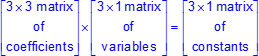• Solution matrix form for inverse matrices
• Ex: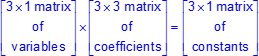• Augmented matrices
• Matrix form for augmented matrices
• Ex: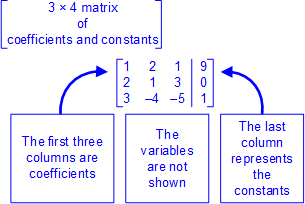• Substitution
• Elimination
• Special cases
• All variables are eliminated
• Infinite number of solutions – remaining constants yield a true statement
• No solutions – remaining constants yield a false statement
• Calculator gives an error message
• Infinite number of solutions – last row is all zeros and yields 0 = 0, which is a true statement.
• Ex: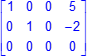• No solutions – last row is not all zeros and ends up 0 = 1, which is not a true statement.
• Ex: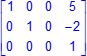Note(s):

• Algebra I solved systems of two linear equations in two variables using graphs, tables, and algebraic methods.
• Various mathematical process standards will be applied to this student expectation as appropriate.
• TxCCRS:
• II. Algebraic Reasoning
• A1 – Explain and differentiate between expressions and equations using words such as “solve,” “evaluate,” and “simplify.”
• C1 – Recognize and use algebraic (field) properties, concepts, procedures, and algorithms to solve equations, inequalities, and systems of linear equations.
• D1 – Interpret multiple representations of equations and relationships.
• D2 – Translate among multiple representations of equations and relationships.
• VIII. Problem Solving and Reasoning
• IX. Communication and Representation
• X. Connections
2A.3C Solve, algebraically, systems of two equations in two variables consisting of a linear equation and a quadratic equation.
Supporting Standard

Solve

SYSTEMS OF TWO EQUATIONS IN TWO VARIABLES CONSISTING OF A LINEAR EQUATION AND A QUADRATIC EQUATION, ALGEBRAICALLY

Including, but not limited to:

• Two equations in two variables
• One linear equation
• One quadratic equation
• Methods for solving systems of equations consisting of one linear equation and one quadratic equation
• Tables
• Common points on tables
• Graphs
• Identification of possible solutions in terms of points of intersection
• Algebraic methods
• Substitution of linear equation into quadratic
• Solve by factoring
• Solve by quadratic formula
• Solve by completing the square

Note(s):

• Algebra I solved systems of two linear equations in two variables using graphs, tables, and algebraic methods.
• Various mathematical process standards will be applied to this student expectation as appropriate.
• TxCCRS:
• II. Algebraic Reasoning
• A1 – Explain and differentiate between expressions and equations using words such as “solve,” “evaluate,” and “simplify.”
• C1 – Recognize and use algebraic (field) properties, concepts, procedures, and algorithms to solve equations, inequalities, and systems of linear equations.
• D1 – Interpret multiple representations of equations and relationships.
• D2 – Translate among multiple representations of equations and relationships.
• VIII. Problem Solving and Reasoning
• IX. Communication and Representation
• X. Connections
2A.3E Formulate systems of at least two linear inequalities in two variables.
Supporting Standard

Formulate

SYSTEMS OF AT LEAST TWO LINEAR INEQUALITIES IN TWO VARIABLES

Including, but not limited to:

• Systems of linear inequalities in two variables
• Two variables or unknowns
• Two or more inequalities
• Mathematical problem situations
• Graphical interpretation
• Verbal interpretation
• Real-world problem situations represented by systems of inequalities
• Two linear inequalities
• Linear programming problem situations

Note(s):

• Algebra I wrote linear inequalities in two variables given a table of values, a graph, and a verbal description.
• Algebra I solved systems of two linear inequalities in two variables using graphs, tables, and algebraic methods.
• Various mathematical process standards will be applied to this student expectation as appropriate.
• TxCCRS:
• VII. Functions
• C1 – Apply known function models.
• C2 – Develop a function to model a situation.
• VIII. Problem Solving and Reasoning
• IX. Communication and Representation
• X. Connections
2A.3G Determine possible solutions in the solution set of systems of two or more linear inequalities in two variables.
Supporting Standard

Determine

POSSIBLE SOLUTIONS IN THE SOLUTION SET OF SYSTEMS OF TWO OR MORE LINEAR INEQUALITIES IN TWO VARIABLES

Including, but not limited to:

• Method for solving system of inequalities
• Graphical analysis of system
• Graphing of each function
• Solid line for ≤ or ≥
• Dashed line for < or >
• Shading of inequality region for each function
• Methods for solving linear programming problem situations
• Graphical analysis of system
• Graphing of each function
• Shading of inequality region for each function
• Identification of common or feasible region of intersection
• Determination of points of intersection by solving system of equations
• Testing the points of intersection that create the vertices of the feasible region by substituting them into the objective function and determining the appropriate outcome
• Conclusion in terms of the linear programming problem situation
• Representation of the solution as points in the solution region
• Justification of solutions to system of inequalities
• Verbal description
• Tables
• Graphs
• Substitution of solutions into original functions
• Justification of reasonableness of solution in terms of real-world problem situations

Note(s):

• Algebra I solved systems of two linear inequalities in two variables using graphs, tables, and algebraic methods.
• Various mathematical process standards will be applied to this student expectation as appropriate.
• TxCCRS:
• II. Algebraic Reasoning
• C1 – Recognize and use algebraic (field) properties, concepts, procedures, and algorithms to solve equations, inequalities, and systems of linear equations.
• III. Geometric Reasoning
• C1 – Make connections between geometry and algebra.
• VII. Functions
• C1 – Apply known function models.
• C2 – Develop a function to model a situation.
• VIII. Problem Solving and Reasoning
• IX. Communication and Representation
• X. Connections
2A.4 Quadratic and square root functions, equations, and inequalities. The student applies mathematical processes to understand that quadratic and square root functions, equations, and quadratic inequalities can be used to model situations, solve problems, and make predictions. The student is expected to:
2A.4E

Formulate quadratic and square root equations using technology given a table of data.

Supporting Standard

Formulate

QUADRATIC EQUATIONS USING TECHNOLOGY GIVEN A TABLE OF DATA

Including, but not limited to:

• Data collection activities with and without technology
• Data modeled by quadratic functions
• Real-world problem situations
• Real-world problem situations modeled by quadratic functions
• Data tables with at least three data points
• Technology methods
• Transformations of f(x) = x2 and f(x) =• Solving three by three matrix to determine ab, and c for f(x) = ax2 + bx + c

Note(s):

• Algebra I solved quadratic equations having real solutions using tables, graphs, factoring, completing the square, quadratic formula, and technology.
• Algebra I wrote, using technology, quadratic functions that provide a reasonable fit to date to estimate solutions and make predictions for real-world problems.
• Various mathematical process standards will be applied to this student expectation as appropriate.
• TxCCRS:
• VII. Functions
• B1 – Understand and analyze features of a function.
• B2 – Algebraically construct and analyze new functions.
• C2 – Develop a function to model a situation.
• VIII. Problem Solving and Reasoning
• IX. Communication and Representation
• X. Connections
2A.4F

Solve quadratic and square root equations.

Solve

Including, but not limited to:

• Methods for solving quadratic equations with and without technology
• Tables
• Zeros – the value(s) of x such that the y value of the relation equals zero
• Domain values with equal range values
• Graphs
• x-intercept(s) – x coordinate of a point at which the relation crosses the x-axis, meaning the y coordinate equals zero, (x, 0)
• Zeros – the value(s) of x such that the y value of the relation equals zero
• Algebraic methods
• Factoring
• Solving equations by taking square roots
• Solving quadratic equations using absolute value
•• Completing the square
• Quadratic formula, x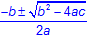• The discriminant, b2 – 4ac, can be used to analyze types of solutions for quadratic equations.
• b2 – 4ac = 0, one rational double root
• b2 – 4ac > 0 and perfect square, two rational roots
• b2 – 4ac > 0 and not perfect square, two irrational roots (conjugates)
• Connections between solutions and roots of quadratic equations to the zeros and x-intercepts of the related function
• Reasonableness of solutions

Note(s):

• Algebra I solved quadratic equations having real solutions using tables, graphs, factoring, completing the square, and the quadratic formula.
• Various mathematical process standards will be applied to this student expectation as appropriate.
• TxCCRS:
• I. Numeric Reasoning
• A2 – Define and give examples of complex numbers.
• B1 – Perform computations with real and complex numbers.
• II. Algebraic Reasoning
• A1 – Explain and differentiate between expressions and equations using words such as “solve,” “evaluate,” and “simplify.”
• C1 – Recognize and use algebraic (field) properties, concepts, procedures, and algorithms to solve equations, inequalities, and systems of linear equations.
• D1 – Interpret multiple representations of equations and relationships.
• D2 – Translate among multiple representations of equations and relationships.
• III. Geometric Reasoning
• C1 – Make connections between geometry and algebra.
• VIII. Problem Solving and Reasoning
• IX. Communication and Representation
• X. Connections
2A.5 Exponential and logarithmic functions and equations. The student applies mathematical processes to understand that exponential and logarithmic functions can be used to model situations and solve problems. The student is expected to:
2A.5B

Formulate exponential and logarithmic equations that model real-world situations, including exponential relationships written in recursive notation.

Supporting Standard

Formulate

EXPONENTIAL EQUATIONS THAT MODEL REAL-WORLD SITUATIONS, INCLUDING EXPONENTIAL RELATIONSHIPS WRITTEN IN RECURSIVE NOTATION

Including, but not limited to:

• Data collection activities with and without technology
• Data modeled by exponential functions
• Exponential growth – an exponential function where b > 1 and as x increases, y increases exponentially
• Exponential decay – an exponential function where 0 < b < 1 and as x increases, y decreases exponentially
• Real-world problem situations
• Real-world problem situations modeled by exponential functions
• Exponential growth
• Exponential decay
• Representations of exponential equations
• Tables/graphs
• Verbal descriptions
• Recursive notation – notation which defines how each term of an iterative rule or formula is related to one or more preceding terms
• Recursive formula: starting value and recursion equation
• Exponential recursive formulas
• Exponential growth: a1 = 5, an = 4an – 1
• Exponential decay: a1 = 64, an =an – 1
• Technology methods
• Transformations of f(x) = bx and y = logbx
• Exponential regression

Note(s):

• Algebra I determined formulas and terms for geometric sequences given in recursive and function notation.
• Algebra II introduces formulating exponential equations.
• Various mathematical process standards will be applied to this student expectation as appropriate.
• TxCCRS:
• VII. Functions
• B1 – Understand and analyze features of a function.
• B2 – Algebraically construct and analyze new functions.
• C2 – Develop a function to model a situation.
• VIII. Problem Solving and Reasoning
• IX. Communication and Representation
• X. Connections
2A.5D

Solve exponential equations of the form y = abx where a is a nonzero real number and b is greater than zero and not equal to one and single logarithmic equations having real solutions.

Solve

EXPONENTIAL EQUATIONS OF THE FORM y = abx WHERE a IS A NONZERO REAL NUMBER AND b IS GREATER THAN ZERO AND NOT EQUAL TO ONE

Including, but not limited to:

• Exponential equation, y = abx
• a – initial value at x = 0
• b – common ratio
• Solving exponential equations
• Application of laws (properties) of exponents
• Real-world problem situations modeled by exponential functions
• Exponential growth
• f(x) = abx, where b > 1
• f(x) = aekx, where k > 0
• Exponential decay
• f(x) = abx, where 0 < b < 1
• f(x) = aekx, where k < 0

Note(s):

• Algebra I applied exponential functions to problem situations using tables, graphs, and the algebraic generalization, f(x) = a • bx.
• Algebra II solves exponential equations algebraically.
• Various mathematical process standards will be applied to this student expectation as appropriate.
• TxCCRS:
• I. Numeric Reasoning
• B1 – Perform computations with real and complex numbers.
• II. Algebraic Reasoning
• A1 – Explain and differentiate between expressions and equations using words such as “solve,” “evaluate,” and “simplify.”
• C1 – Recognize and use algebraic (field) properties, concepts, procedures, and algorithms to solve equations, inequalities, and systems of linear equations.
• D1 – Interpret multiple representations of equations and relationships.
• D2 – Translate among multiple representations of equations and relationships.
• III. Geometric Reasoning
• C1 – Make connections between geometry and algebra.
• VIII. Problem Solving and Reasoning
• IX. Communication and Representation
• X. Connections
2A.6 Cubic, cube root, absolute value and rational functions, equations, and inequalities. The student applies mathematical processes to understand that cubic, cube root, absolute value and rational functions, equations, and inequalities can be used to model situations, solve problems, and make predictions. The student is expected to:
2A.6L Formulate and solve equations involving inverse variation.

Formulate

EQUATIONS INVOLVING INVERSE VARIATION

Including, but not limited to:

• Characteristics of variation
• Constant of variation
• Particular equation to represent variation
• Types of variation
• Direct variation – a relationship between two variables, x (independent) and y (dependent), that always has a constant, unchanged ratio, k, and can be represented by y = kx
• y varies directly as x
• General equation: y = kx
• Connection of direct variation to linear functions
• Inverse variation – a relationship between two variables, x (independent) and y (dependent), that always has a constant, unchanged ratio, k, and can be represented by y =• y varies inversely as x
• General equation: y =• Connection of inverse variation to rational functions
• Real-world problem situations involving variation
• Reasonableness of solutions mathematically and in context of real-world problem situations

Solve

EQUATIONS INVOLVING INVERSE VARIATION

Including, but not limited to:

• Methods for solving variation equations with and without technology
• Graphs
• Algebraic methods
• Solving processes
• Determination of a particular equation to represent the problem
• Direct variation, y = kx
• Inverse variation, y =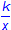• Transformation of equation to solve for unknown
• Justification of solutions with and without technology
• Substitution of solutions into original functions
• Real-world problem situations modeled by rational functions
• Justification of reasonableness of solutions in terms of real-world problem situations or data collections

Note(s):

• Prior grade levels studied direct variation and proportionality.
• Algebra II introduces inverse variation and its applications in problem situations.
• Precalculus will continue to investigate rational functions.
• Various mathematical process standards will be applied to this student expectation as appropriate.
• TxCCRS:
• I. Numeric Reasoning
• B1 – Perform computations with real and complex numbers.
• II. Algebraic Reasoning
• A1 – Explain and differentiate between expressions and equations using words such as “solve,” “evaluate,” and “simplify.”
• C1 – Recognize and use algebraic (field) properties, concepts, procedures, and algorithms to solve equations, inequalities, and systems of linear equations.
• D1 – Interpret multiple representations of equations and relationships.
• D2 – Translate among multiple representations of equations and relationships.
• III. Geometric Reasoning
• C1 – Make connections between geometry and algebra.
• VII. Functions
• B2 – Algebraically construct and analyze new functions.
• C2 – Develop a function to model a situation.
• VIII. Problem Solving and Reasoning
• IX. Communication and Representation
• X. Connections
2A.8 Data. The student applies mathematical processes to analyze data, select appropriate models, write corresponding functions, and make predictions. The student is expected to:
2A.8C Predict and make decisions and critical judgments from a given set of data using linear, quadratic, and exponential models.

Predict, Make

DECISIONS AND CRITICAL JUDGMENTS FROM A GIVEN SET OF DATA USING LINEAR, QUADRATIC, AND EXPONENTIAL MODELS

Including, but not limited to:

• Mathematical and real-world problem situations modeled by linear, quadratic, and exponential functions and equations
• Predictions, decisions, and critical judgments from function models
• Justification of reasonableness of solutions in terms of mathematical and real-world problem situations
• Mathematical justification
• Substitution in original problem
• Justification for predictions using the coefficient of determination, r2

Note(s):

• Algebra I introduced the linear, quadratic, and exponential functions.
• Algebra I introduced the correlation coefficient as a measure of the strength of linear association.
• Algebra I applied linear, quadratic, and exponential functions to model and make predictions in real-world problem situations.
• Various mathematical process standards will be applied to this student expectation as appropriate.
• TxCCRS:
• I. Numeric Reasoning
• B1 – Perform computations with real and complex numbers.
• II. Algebraic Reasoning
• A1 – Explain and differentiate between expressions and equations using words such as “solve,” “evaluate,” and “simplify.”
• B1 – Recognize and use algebraic (field) properties, concepts, procedures, and algorithms to combine, transform, and evaluate expressions (e.g. polynomials, radicals, rational expressions).
• C1 – Recognize and use algebraic (field) properties, concepts, procedures, and algorithms to solve equations, inequalities, and systems of linear equations.
• D1 – Interpret multiple representations of equations and relationships.
• D2 – Translate among multiple representations of equations and relationships.
• III. Geometric Reasoning
• C1 – Make connections between geometry and algebra.
• VII. Functions
• A2 – Recognize and distinguish between different types of functions.
• B1 – Understand and analyze features of a function.
• B2 – Algebraically construct and analyze new functions.
• VIII. Problem Solving and Reasoning
• IX. Communication and Representation
• X. Connections